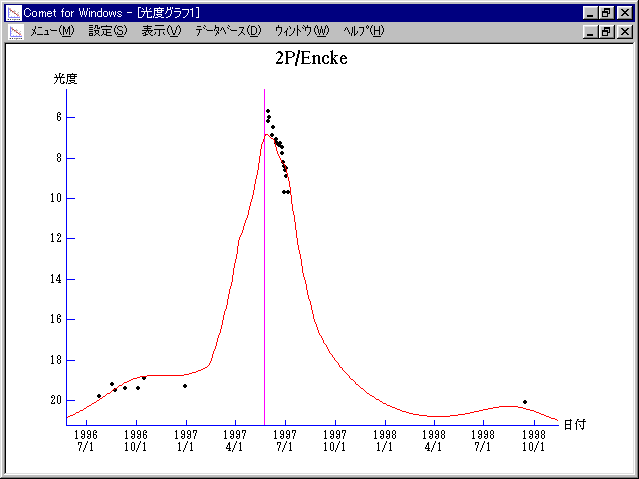# 2P/Encke (1997)###Orbital Elements

```   The following improved orbital elements by Kenji Muraoka,
are from 250 observations 1982 to 1998, including 9 Planets,
Moon and 5 minor planets perturbations and non-gravitational
effect of style II.  The mean residual is +/- 0.83 arc seconds.

Epoch  =  1997 June  1.0  TT       JDT = 2450600.5
T  =  1997 May  23.59866       +/- 0.00020 (m.e.) TT
Peri. =  186.27254                +/- 0.00007
Node  =  334.72093                +/- 0.00006   (2000.0)
Incl. =   11.92936                +/- 0.00001
q  =    0.3313956              +/- 0.0000002 AU
e  =    0.8500137              +/- 0.0000001
a  =    2.2095057              +/- 0.0000006 AU
n  =    0.30009682             +/- 0.00000012
P  =    3.284                  +/- 0.0000014  years
A1  =   -0.009                  +/- 0.003
A2  =   -0.00132                +/- 0.00002
```

###Finding Charts1996 July 6 - 1997 June 261997 June 11 - 1997 July 161997 July 11 - 1998 Mar. 18

###Magnitudes Graph

```        m1 = 15.0 + 5 log d +  5.0 log r  [    ,-100]  (             - 1997 Feb. 12)
m1 =  9.8 + 5 log d + 25   log r  [-100, -45]  (1997 Feb. 12 - 1997 Apr.  8)
m1 = 10.3 + 5 log d +  7.0 log r  [ -45,  20]  (1997 Apr.  8 - 1997 June 12)
m1 = 12.3 + 5 log d + 15.7 log r  [  20, 100]  (1997 June 12 - 1997 Aug. 31)
m1 = 15.0 + 5 log d +  5.0 log r  [ 100,    ]  (1997 Aug. 31 -             )
```##### The orbital elements are calculated by Kenji Muraoka. The charts are made with StellaNavigator Ver.2.0 for Windows (AstroArts / ASCII). The magnitudes graphs are made with Comet for Windows.